Comparison
Comparison close
Results

MEASURAND : Relative error of a gas flow meter
GAS FLOW RATE : 2 m3/h to 100 m3/h
TRANSFER STANDARD : A rotary gas meter (see Section 3 of the Final Report)

The curve of the key comparison reference value is added on the graph showing the values xi and UiS obtained by this participant.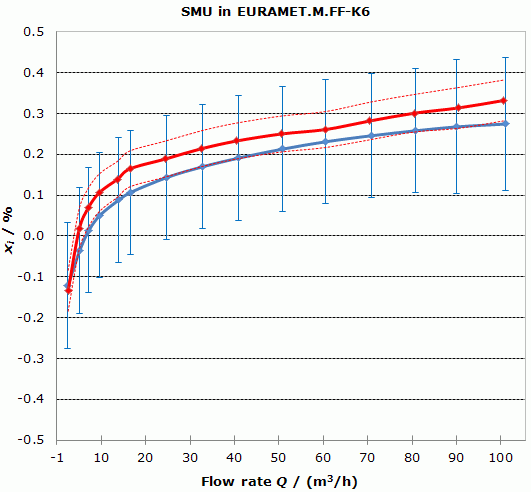The solid blue curve represents the participant's results, xi, with expanded uncertainty bars (k = 2), UiS.
The solid red curve represents the key comparison reference value.
The two red dash curves correspond to (xR + UR) and (xR - UR), where UR is the expanded uncertainty (k = 2) of xR.

MEASURAND : Relative error of a gas flow meter
GAS FLOW RATE : 2 m3/h to 100 m3/h
TRANSFER STANDARD : A rotary gas meter (see Section 3 of the Final Report)

The curve of the key comparison reference value is added on the graph showing the values xi and UiS obtained by this participant.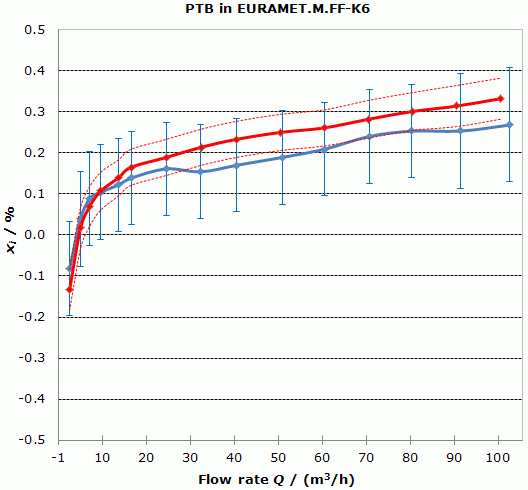The solid blue curve represents the participant's results, xi, with expanded uncertainty bars (k = 2), UiS.
The solid red curve represents the key comparison reference value.
The two red dash curves correspond to (xR + UR) and (xR - UR), where UR is the expanded uncertainty (k = 2) of xR.

MEASURAND : Relative error of a gas flow meter
GAS FLOW RATE : 2 m3/h to 100 m3/h
TRANSFER STANDARD : A rotary gas meter (see Section 3 of the Final Report)

The curve of the key comparison reference value is added on the graph showing the values xi and UiS obtained by this participant.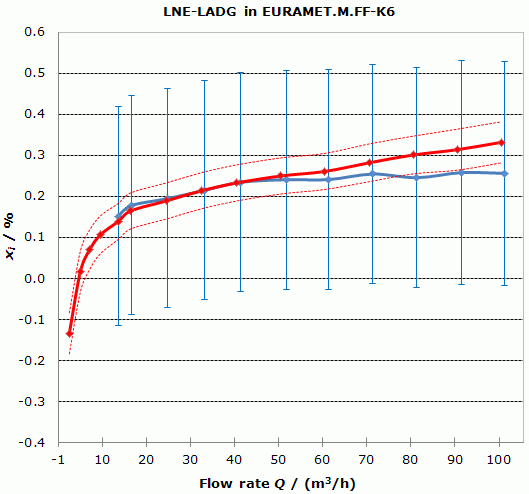The solid blue curve represents the participant's results, xi, with expanded uncertainty bars (k = 2), UiS.
The solid red curve represents the key comparison reference value.
The two red dash curves correspond to (xR + UR) and (xR - UR), where UR is the expanded uncertainty (k = 2) of xR.

MEASURAND : Relative error of a gas flow meter
GAS FLOW RATE : 2 m3/h to 100 m3/h
TRANSFER STANDARD : A rotary gas meter (see Section 3 of the Final Report)

The curve of the key comparison reference value is added on the graph showing the values xi and UiS obtained by this participant.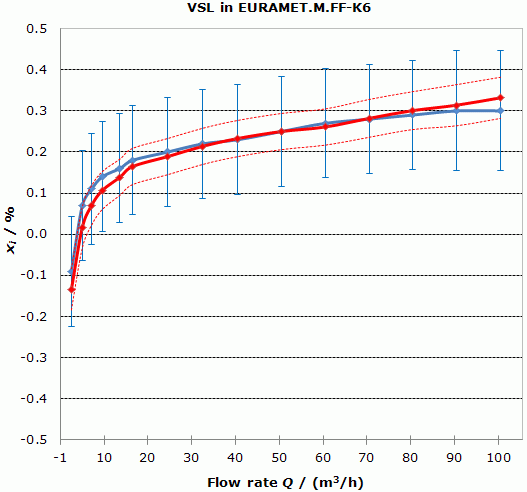The solid blue curve represents the participant's results, xi, with expanded uncertainty bars (k = 2), UiS.
The solid red curve represents the key comparison reference value.
The two red dash curves correspond to (xR + UR) and (xR - UR), where UR is the expanded uncertainty (k = 2) of xR.

MEASURAND : Relative error of a gas flow meter
GAS FLOW RATE : 2 m3/h to 100 m3/h
TRANSFER STANDARD : A rotary gas meter (see Section 3 of the Final Report)

The curve of the key comparison reference value is added on the graph showing the values xi and UiS obtained by this participant.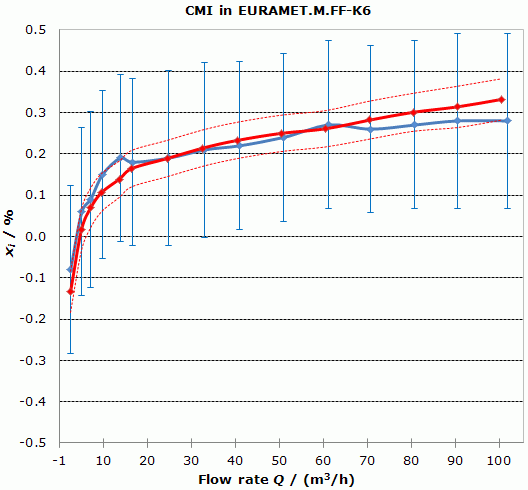The solid blue curve represents the participant's results, xi, with expanded uncertainty bars (k = 2), UiS.
The solid red curve represents the key comparison reference value.
The two red dash curves correspond to (xR + UR) and (xR - UR), where UR is the expanded uncertainty (k = 2) of xR.

MEASURAND : Relative error of a gas flow meter
GAS FLOW RATE : 2 m3/h to 100 m3/h
TRANSFER STANDARD : A rotary gas meter (see Section 3 of the Final Report)

The curve of the key comparison reference value is added on the graph showing the values xi and UiS obtained by this participant.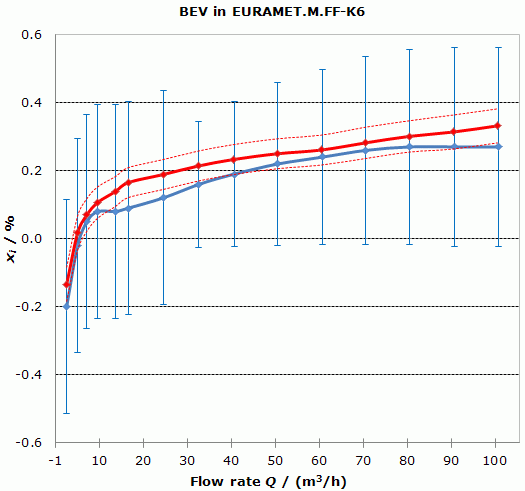The solid blue curve represents the participant's results, xi, with expanded uncertainty bars (k = 2), UiS.
The solid red curve represents the key comparison reference value.
The two red dash curves correspond to (xR + UR) and (xR - UR), where UR is the expanded uncertainty (k = 2) of xR.

MEASURAND : Relative error of a gas flow meter
GAS FLOW RATE : 2 m3/h to 100 m3/h
TRANSFER STANDARD : A rotary gas meter (see Section 3 of the Final Report)

The curve of the key comparison reference value is added on the graph showing the values xi and UiS obtained by this participant.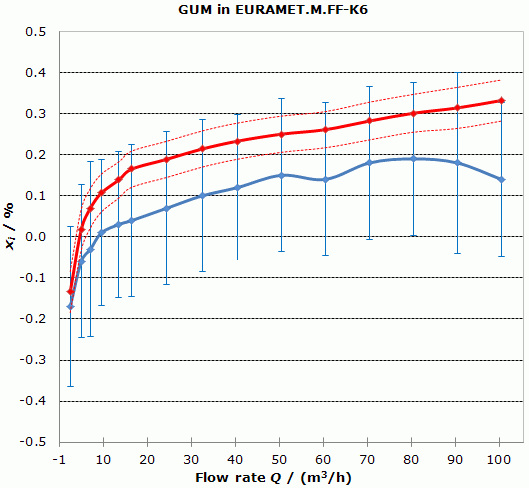The solid blue curve represents the participant's results, xi, with expanded uncertainty bars (k = 2), UiS.
The solid red curve represents the key comparison reference value.
The two red dash curves correspond to (xR + UR) and (xR - UR), where UR is the expanded uncertainty (k = 2) of xR.

MEASURAND : Relative error of a gas flow meter
GAS FLOW RATE : 2 m3/h to 100 m3/h
TRANSFER STANDARD : A rotary gas meter (see Section 3 of the Final Report)

The curve of the key comparison reference value is added on the graph showing the values xi and UiS obtained by this participant.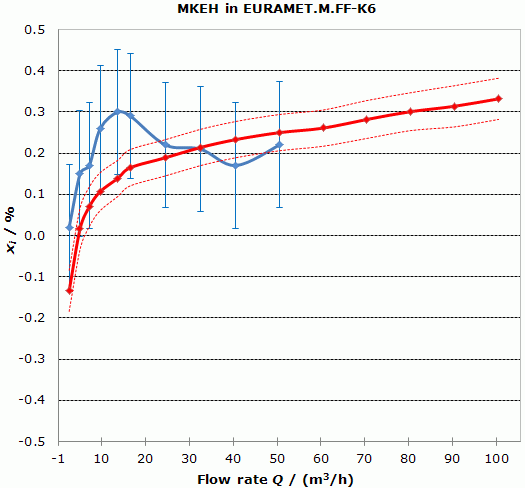The solid blue curve represents the participant's results, xi, with expanded uncertainty bars (k = 2), UiS.
The solid red curve represents the key comparison reference value.
The two red dash curves correspond to (xR + UR) and (xR - UR), where UR is the expanded uncertainty (k = 2) of xR.

MEASURAND : Relative error of a gas flow meter
GAS FLOW RATE : 2 m3/h to 100 m3/h
TRANSFER STANDARD : A rotary gas meter (see Section 3 of the Final Report)

The curve of the key comparison reference value is added on the graph showing the values xi and UiS obtained by this participant.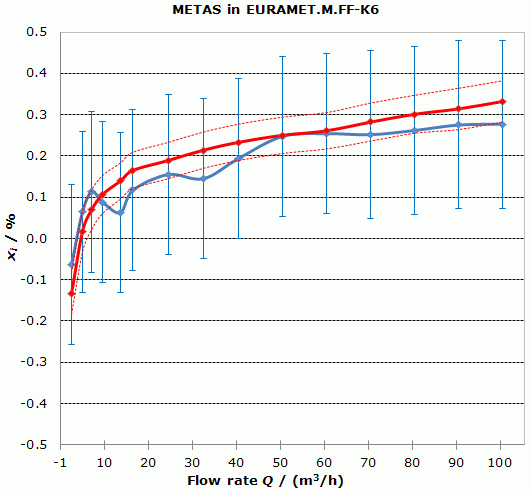The solid blue curve represents the participant's results, xi, with expanded uncertainty bars (k = 2), UiS.
The solid red curve represents the key comparison reference value.
The two red dash curves correspond to (xR + UR) and (xR - UR), where UR is the expanded uncertainty (k = 2) of xR.

MEASURAND : Relative error of a gas flow meter
GAS FLOW RATE : 2 m3/h to 100 m3/h
TRANSFER STANDARD : A rotary gas meter (see Section 3 of the Final Report)

The curve of the key comparison reference value is added on the graph showing the values xi and UiS obtained by this participant.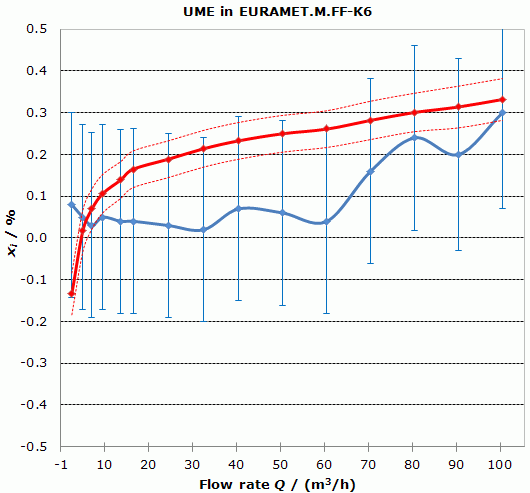The solid blue curve represents the participant's results, xi, with expanded uncertainty bars (k = 2), UiS.
The solid red curve represents the key comparison reference value.
The two red dash curves correspond to (xR + UR) and (xR - UR), where UR is the expanded uncertainty (k = 2) of xR.

MEASURAND : Relative error of a gas flow meter
GAS FLOW RATE : 2 m3/h to 100 m3/h
TRANSFER STANDARD : A rotary gas meter (see Section 3 of the Final Report)

The curve of the key comparison reference value is added on the graph showing the values xi and UiS obtained by this participant.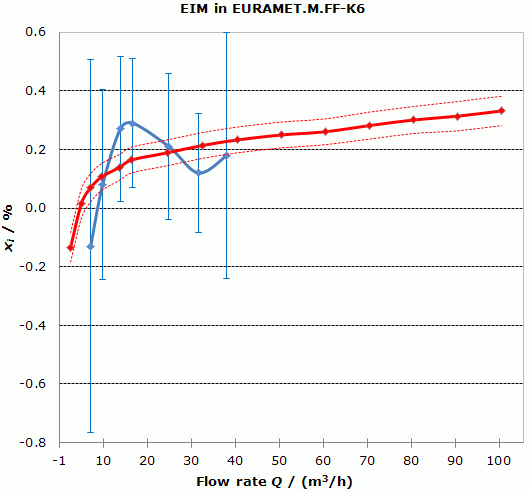The solid blue curve represents the participant's results, xi, with expanded uncertainty bars (k = 2), UiS.
The solid red curve represents the key comparison reference value.
The two red dash curves correspond to (xR + UR) and (xR - UR), where UR is the expanded uncertainty (k = 2) of xR.

MEASURAND : Relative error of a gas flow meter
GAS FLOW RATE : 2 m3/h to 100 m3/h
TRANSFER STANDARD : A rotary gas meter (see Section 3 of the Final Report)

The curve of the key comparison reference value is added on the graph showing the values xi and UiS obtained by this participant.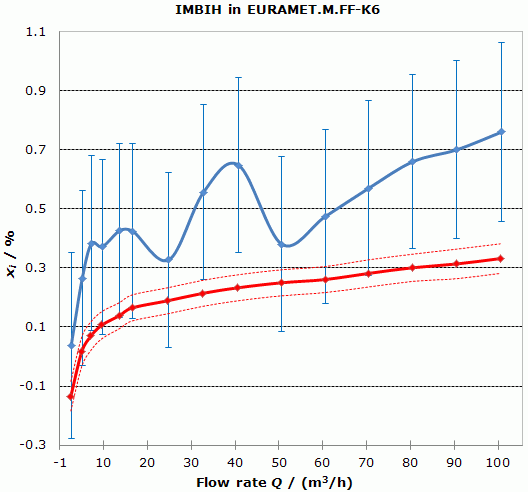The solid blue curve represents the participant's results, xi, with expanded uncertainty bars (k = 2), UiS.
The solid red curve represents the key comparison reference value.
The two red dash curves correspond to (xR + UR) and (xR - UR), where UR is the expanded uncertainty (k = 2) of xR.

MEASURAND : Relative error of a gas flow meter
GAS FLOW RATE : 2 m3/h to 100 m3/h
TRANSFER STANDARD : A rotary gas meter (see Section 3 of the Final Report)

The curve of the key comparison reference value is added on the graph showing the values xi and UiS obtained by this participant.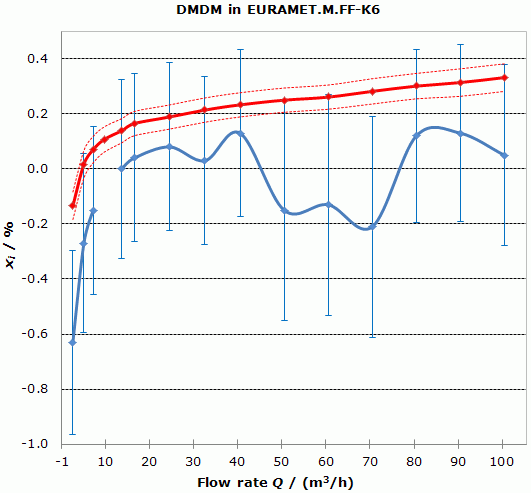The solid blue curve represents the participant's results, xi, with expanded uncertainty bars (k = 2), UiS.
The solid red curve represents the key comparison reference value.
The two red dash curves correspond to (xR + UR) and (xR - UR), where UR is the expanded uncertainty (k = 2) of xR.

MEASURAND : Relative error of a gas flow meter
GAS FLOW RATE : 2 m3/h to 100 m3/h
TRANSFER STANDARD : A rotary gas meter (see Section 3 of the Final Report)

The curve of the key comparison reference value is added on the graph showing the values xi and UiS obtained by this participant.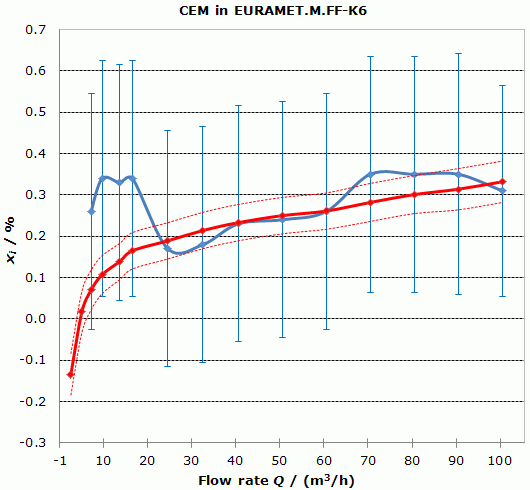The solid blue curve represents the participant's results, xi, with expanded uncertainty bars (k = 2), UiS.
The solid red curve represents the key comparison reference value.
The two red dash curves correspond to (xR + UR) and (xR - UR), where UR is the expanded uncertainty (k = 2) of xR.

MEASURAND : Relative error of a gas flow meter
GAS FLOW RATE : 2 m3/h to 100 m3/h
TRANSFER STANDARD : A rotary gas meter (see Section 3 of the Final Report)

The curve of the key comparison reference value is added on the graph showing the values xi and UiS obtained by this participant.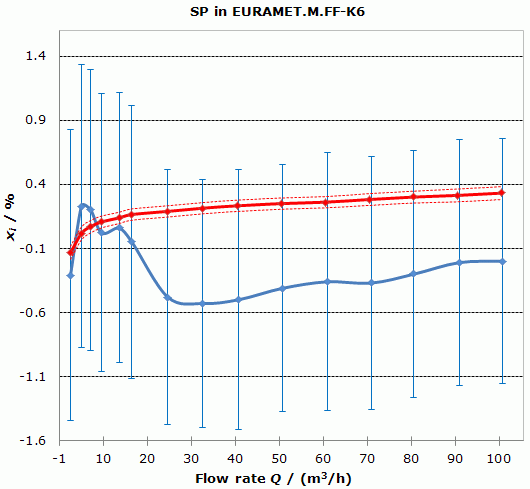The solid blue curve represents the participant's results, xi, with expanded uncertainty bars (k = 2), UiS.
The solid red curve represents the key comparison reference value.
The two red dash curves correspond to (xR + UR) and (xR - UR), where UR is the expanded uncertainty (k = 2) of xR.

Comparison
Comparison close
Results

MEASURAND : Relative error of a gas flow meter
GAS FLOW RATE : 2 m3/h to 100 m3/h
TRANSFER STANDARD : A rotary gas meter (see Section 3 of the Final Report)

Equivalence is demonstrated by providing the Di and Eni values for one participating laboratory over the complete range of flow rates. The curve of the key comparison reference value is added on the graph showing the values xi and UiS obtained by this participant.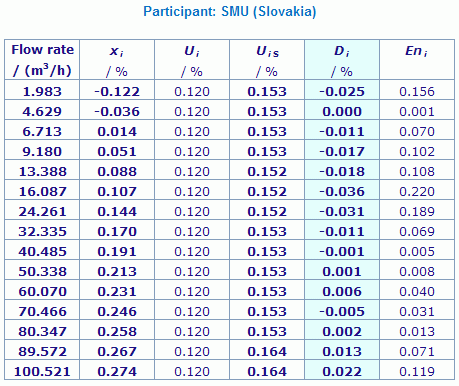Results are presented under A4 printable format in Summary Results (PDF file).

Unless otherwise stated, in the final numbers presented here, rounding has been applied according to ISO 80000-1:2009 Annex B Rule B.

MEASURAND : Relative error of a gas flow meter
GAS FLOW RATE : 2 m3/h to 100 m3/h
TRANSFER STANDARD : A rotary gas meter (see Section 3 of the Final Report)

Equivalence is demonstrated by providing the Di and Eni values for one participating laboratory over the complete range of flow rates. The curve of the key comparison reference value is added on the graph showing the values xi and UiS obtained by this participant.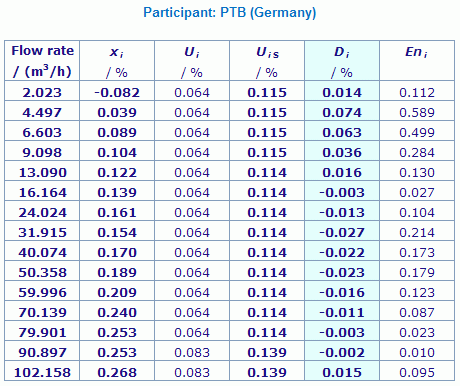Results are presented under A4 printable format in Summary Results (PDF file).

Unless otherwise stated, in the final numbers presented here, rounding has been applied according to ISO 80000-1:2009 Annex B Rule B.

MEASURAND : Relative error of a gas flow meter
GAS FLOW RATE : 2 m3/h to 100 m3/h
TRANSFER STANDARD : A rotary gas meter (see Section 3 of the Final Report)

Equivalence is demonstrated by providing the Di and Eni values for one participating laboratory over the complete range of flow rates. The curve of the key comparison reference value is added on the graph showing the values xi and UiS obtained by this participant.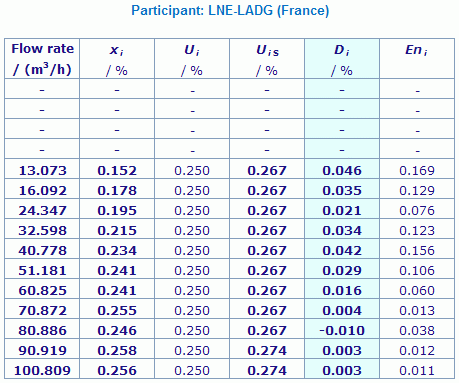Results are presented under A4 printable format in Summary Results (PDF file).

Unless otherwise stated, in the final numbers presented here, rounding has been applied according to ISO 80000-1:2009 Annex B Rule B.

MEASURAND : Relative error of a gas flow meter
GAS FLOW RATE : 2 m3/h to 100 m3/h
TRANSFER STANDARD : A rotary gas meter (see Section 3 of the Final Report)

Equivalence is demonstrated by providing the Di and Eni values for one participating laboratory over the complete range of flow rates. The curve of the key comparison reference value is added on the graph showing the values xi and UiS obtained by this participant.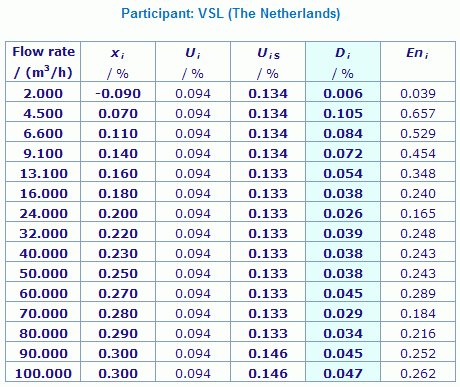Results are presented under A4 printable format in Summary Results (PDF file).

Unless otherwise stated, in the final numbers presented here, rounding has been applied according to ISO 80000-1:2009 Annex B Rule B.

MEASURAND : Relative error of a gas flow meter
GAS FLOW RATE : 2 m3/h to 100 m3/h
TRANSFER STANDARD : A rotary gas meter (see Section 3 of the Final Report)

Equivalence is demonstrated by providing the Di and Eni values for one participating laboratory over the complete range of flow rates. The curve of the key comparison reference value is added on the graph showing the values xi and UiS obtained by this participant.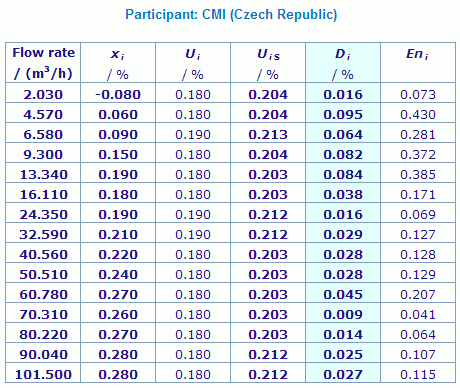Results are presented under A4 printable format in Summary Results (PDF file).

Unless otherwise stated, in the final numbers presented here, rounding has been applied according to ISO 80000-1:2009 Annex B Rule B.

MEASURAND : Relative error of a gas flow meter
GAS FLOW RATE : 2 m3/h to 100 m3/h
TRANSFER STANDARD : A rotary gas meter (see Section 3 of the Final Report)

Equivalence is demonstrated by providing the Di and Eni values for one participating laboratory over the complete range of flow rates. The curve of the key comparison reference value is added on the graph showing the values xi and UiS obtained by this participant.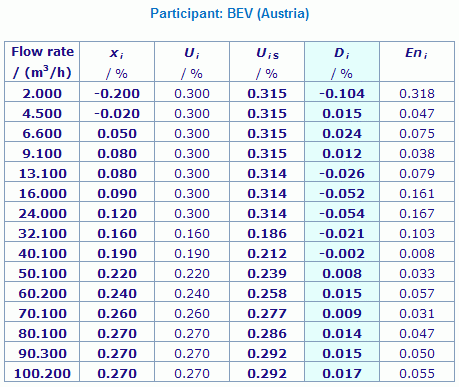Results are presented under A4 printable format in Summary Results (PDF file).

Unless otherwise stated, in the final numbers presented here, rounding has been applied according to ISO 80000-1:2009 Annex B Rule B.

MEASURAND : Relative error of a gas flow meter
GAS FLOW RATE : 2 m3/h to 100 m3/h
TRANSFER STANDARD : A rotary gas meter (see Section 3 of the Final Report)

Equivalence is demonstrated by providing the Di and Eni values for one participating laboratory over the complete range of flow rates. The curve of the key comparison reference value is added on the graph showing the values xi and UiS obtained by this participant.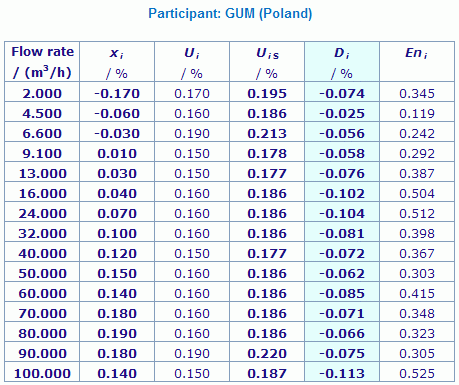Results are presented under A4 printable format in Summary Results (PDF file).

Unless otherwise stated, in the final numbers presented here, rounding has been applied according to ISO 80000-1:2009 Annex B Rule B.

MEASURAND : Relative error of a gas flow meter
GAS FLOW RATE : 2 m3/h to 100 m3/h
TRANSFER STANDARD : A rotary gas meter (see Section 3 of the Final Report)

Equivalence is demonstrated by providing the Di and Eni values for one participating laboratory over the complete range of flow rates. The curve of the key comparison reference value is added on the graph showing the values xi and UiS obtained by this participant.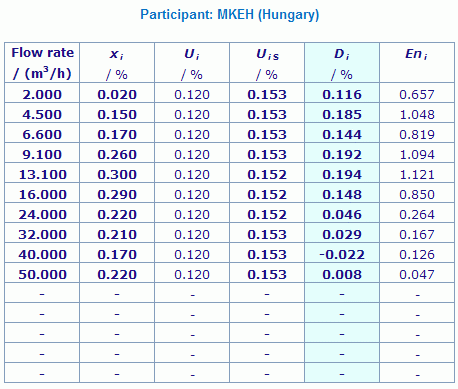Results are presented under A4 printable format in Summary Results (PDF file).

Unless otherwise stated, in the final numbers presented here, rounding has been applied according to ISO 80000-1:2009 Annex B Rule B.

MEASURAND : Relative error of a gas flow meter
GAS FLOW RATE : 2 m3/h to 100 m3/h
TRANSFER STANDARD : A rotary gas meter (see Section 3 of the Final Report)

Equivalence is demonstrated by providing the Di and Eni values for one participating laboratory over the complete range of flow rates. The curve of the key comparison reference value is added on the graph showing the values xi and UiS obtained by this participant.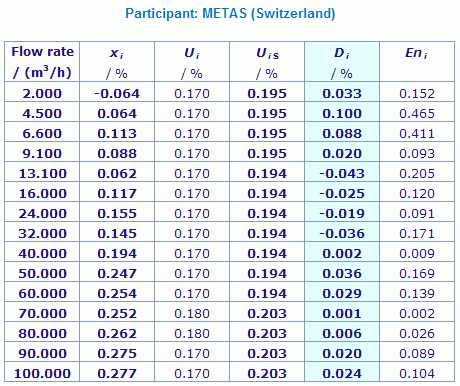Results are presented under A4 printable format in Summary Results (PDF file).

Unless otherwise stated, in the final numbers presented here, rounding has been applied according to ISO 80000-1:2009 Annex B Rule B.

MEASURAND : Relative error of a gas flow meter
GAS FLOW RATE : 2 m3/h to 100 m3/h
TRANSFER STANDARD : A rotary gas meter (see Section 3 of the Final Report)

Equivalence is demonstrated by providing the Di and Eni values for one participating laboratory over the complete range of flow rates. The curve of the key comparison reference value is added on the graph showing the values xi and UiS obtained by this participant.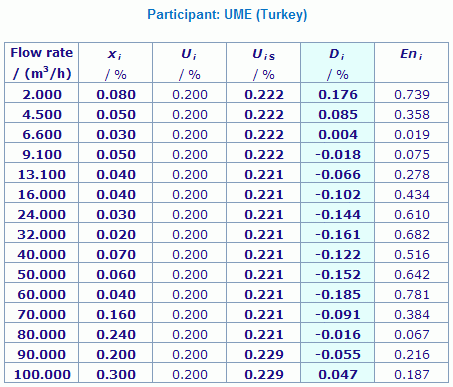Results are presented under A4 printable format in Summary Results (PDF file).

Unless otherwise stated, in the final numbers presented here, rounding has been applied according to ISO 80000-1:2009 Annex B Rule B.

MEASURAND : Relative error of a gas flow meter
GAS FLOW RATE : 2 m3/h to 100 m3/h
TRANSFER STANDARD : A rotary gas meter (see Section 3 of the Final Report)

Equivalence is demonstrated by providing the Di and Eni values for one participating laboratory over the complete range of flow rates. The curve of the key comparison reference value is added on the graph showing the values xi and UiS obtained by this participant.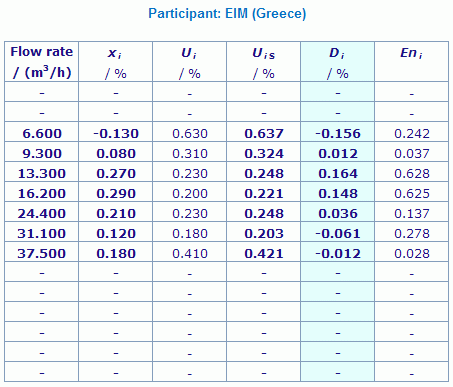Results are presented under A4 printable format in Summary Results (PDF file).

Unless otherwise stated, in the final numbers presented here, rounding has been applied according to ISO 80000-1:2009 Annex B Rule B.

MEASURAND : Relative error of a gas flow meter
GAS FLOW RATE : 2 m3/h to 100 m3/h
TRANSFER STANDARD : A rotary gas meter (see Section 3 of the Final Report)

Equivalence is demonstrated by providing the Di and Eni values for one participating laboratory over the complete range of flow rates. The curve of the key comparison reference value is added on the graph showing the values xi and UiS obtained by this participant.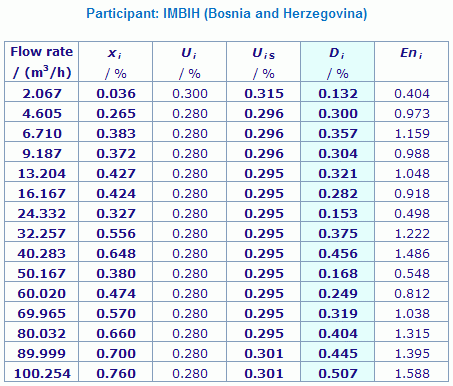Results are presented under A4 printable format in Summary Results (PDF file).

Unless otherwise stated, in the final numbers presented here, rounding has been applied according to ISO 80000-1:2009 Annex B Rule B.

MEASURAND : Relative error of a gas flow meter
GAS FLOW RATE : 2 m3/h to 100 m3/h
TRANSFER STANDARD : A rotary gas meter (see Section 3 of the Final Report)

Equivalence is demonstrated by providing the Di and Eni values for one participating laboratory over the complete range of flow rates. The curve of the key comparison reference value is added on the graph showing the values xi and UiS obtained by this participant.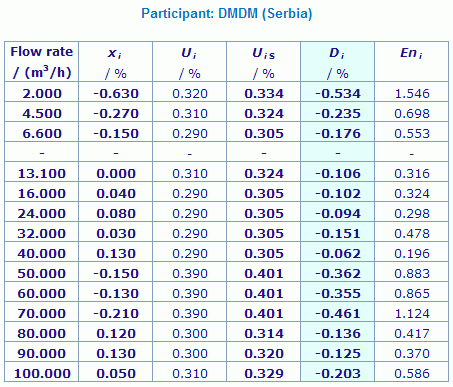Results are presented under A4 printable format in Summary Results (PDF file).

Unless otherwise stated, in the final numbers presented here, rounding has been applied according to ISO 80000-1:2009 Annex B Rule B.

MEASURAND : Relative error of a gas flow meter
GAS FLOW RATE : 2 m3/h to 100 m3/h
TRANSFER STANDARD : A rotary gas meter (see Section 3 of the Final Report)

Equivalence is demonstrated by providing the Di and Eni values for one participating laboratory over the complete range of flow rates. The curve of the key comparison reference value is added on the graph showing the values xi and UiS obtained by this participant.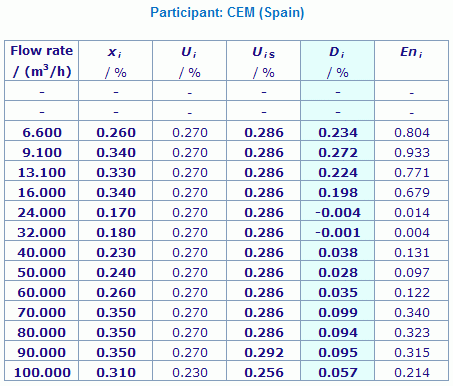Results are presented under A4 printable format in Summary Results (PDF file).

Unless otherwise stated, in the final numbers presented here, rounding has been applied according to ISO 80000-1:2009 Annex B Rule B.

MEASURAND : Relative error of a gas flow meter
GAS FLOW RATE : 2 m3/h to 100 m3/h
TRANSFER STANDARD : A rotary gas meter (see Section 3 of the Final Report)

Equivalence is demonstrated by providing the Di and Eni values for one participating laboratory over the complete range of flow rates. The curve of the key comparison reference value is added on the graph showing the values xi and UiS obtained by this participant.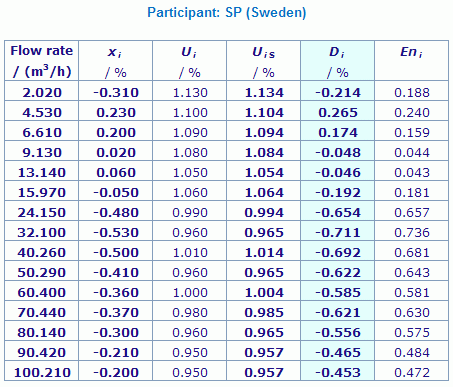Results are presented under A4 printable format in Summary Results (PDF file).

Unless otherwise stated, in the final numbers presented here, rounding has been applied according to ISO 80000-1:2009 Annex B Rule B.

Comparison
Comparison close
RMO comparison
 Metrology area, Sub-field Mass and related quantities, Fluid Flow Description Low pressure gas flow Time of measurements 2010 - 2011 Status Approved for equivalence
 Final Reports of the comparisons References Measurand Volume (m3) of air at low pressure in range of flow 2 m3/h to 100 m3/h Parameters Temperature of air: 15 °C to 25 °CAmbient humidity: 25 % to 75 %Ambient atmospheric pressure: 86 kPa to 106 kPa Transfer device Rotary gas meter Actaris Delta 2050 S-Flow G65
 Comparison type Key Comparison Consultative Committee CCM (Consultative Committee for Mass and Related Quantities) Conducted by EURAMET (European Association of National Metrology Institutes) RMO Internal Identifier EURAMET Project No 1180 Comments Results published on 22 December 2014 EURAMET.M.FF-K6 results are linked to those of CCM.FF-K6.2011 Low pressure gas flow (presented per participating laboratory): Pilot institute CMI Czech Metrology Institute Czechia Contact person Miroslava Benkova +420 734 877 960
First Name Last Name
wwww@ww.www +356719836 Institute 1 Institute 1 Khmelnitskiy
Pilot laboratory
CMI

Czech Metrology Institute, Czechia, EURAMET

 BEV Bundesamt für Eich- und Vermessungswesen, Austria, EURAMET
 CEM Centro Español de Metrologia, Spain, EURAMET
 DMDM Directorate of Measures and Precious Metals, Serbia, EURAMET
 EIM Hellenic Institute of Metrology, Greece, EURAMET
 GUM Glowny Urzad Miar, Central Office of Measures, Poland, EURAMET
 IMBIH Institute of Metrology of Bosnia and Herzegovina, Bosnia and Herzegovina, EURAMET
 LNE-LADG Laboratoire Associé de Débitmétrie Gazeuse, France, EURAMET
 METAS Federal Institute of Metrology, Switzerland, EURAMET
 PTB Physikalisch-Technische Bundesanstalt, Germany, EURAMET
 SMU Slovensky Metrologicky Ustav, Slovakia, EURAMET
 UME TÜBITAK Ulusal Metroloji Enstitüsü, Türkiye, EURAMET
 VSL VSL, Netherlands, EURAMET
Comparison
Comparison close
Results

MEASURAND : Relative error of a gas flow meter
GAS FLOW RATE : 2 m3/h to 100 m3/h
TRANSFER STANDARD : A rotary gas meter (see Section 3 of the Final Report)

 The results of EURAMET.M.FF-K6 are linked to those of CCM.FF-K6.2011 through the common participation of SMU, PTB and LNE-LADG in both key comparisons (see Section 6 of the EURAMET.M.FF-K6 Final Report). The computation of the CCM.FF-K6.2011 key comparison reference value, xR, and of its standard uncertainty, uR, is explained in Section 6 of the CCM.FF-K6.2011 Final Report.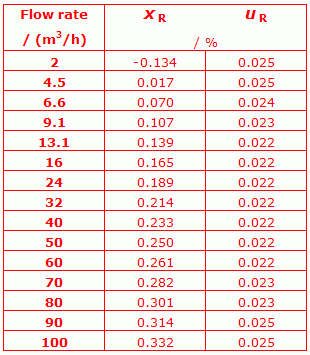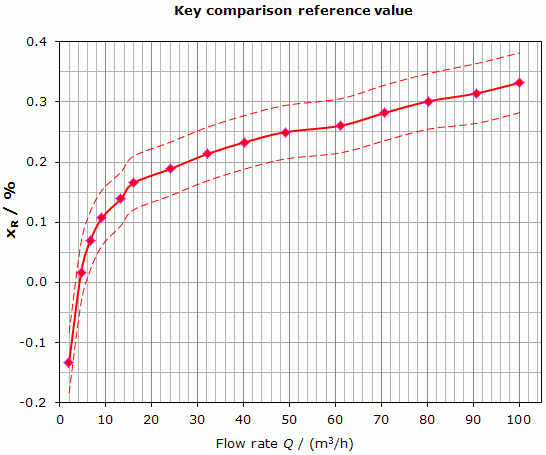The two red dash curves correspond to (xR + UR) and (xR - UR), where UR is the expanded uncertainty (k = 2) of xR.
 The degree of equivalence of laboratory i with respect to the CCM.FF-K6.2011 key comparison reference value is given by a pair of terms: Di and its expanded uncertainty (k = 2), computed as explained in Section 6 of the EURAMET.M.FF-K6 Final Report. The normalized degrees of equivalence Eni are also computed according to equation 17 on page 16 of the same Final Report.
 No pair-wise degrees of equivalence are computed for this key comparison.

MEASURAND : Relative error of a gas flow meter
GAS FLOW RATE : 2 m3/h to 100 m3/h
TRANSFER STANDARD : A rotary gas meter (see Section 3 of the Final Report)

 The results of EURAMET.M.FF-K6 are linked to those of CCM.FF-K6.2011 through the common participation of SMU, PTB and LNE-LADG in both key comparisons (see Section 6 of the EURAMET.M.FF-K6 Final Report). The computation of the CCM.FF-K6.2011 key comparison reference value, xR, and of its standard uncertainty, uR, is explained in Section 6 of the CCM.FF-K6.2011 Final Report.The two red dash curves correspond to (xR + UR) and (xR - UR), where UR is the expanded uncertainty (k = 2) of xR.
 The degree of equivalence of laboratory i with respect to the CCM.FF-K6.2011 key comparison reference value is given by a pair of terms: Di and its expanded uncertainty (k = 2), computed as explained in Section 6 of the EURAMET.M.FF-K6 Final Report. The normalized degrees of equivalence Eni are also computed according to equation 17 on page 16 of the same Final Report.
 No pair-wise degrees of equivalence are computed for this key comparison.

MEASURAND : Relative error of a gas flow meter
GAS FLOW RATE : 2 m3/h to 100 m3/h
TRANSFER STANDARD : A rotary gas meter (see Section 3 of the Final Report)

 The results of EURAMET.M.FF-K6 are linked to those of CCM.FF-K6.2011 through the common participation of SMU, PTB and LNE-LADG in both key comparisons (see Section 6 of the EURAMET.M.FF-K6 Final Report). The computation of the CCM.FF-K6.2011 key comparison reference value, xR, and of its standard uncertainty, uR, is explained in Section 6 of the CCM.FF-K6.2011 Final Report.The two red dash curves correspond to (xR + UR) and (xR - UR), where UR is the expanded uncertainty (k = 2) of xR.
 The degree of equivalence of laboratory i with respect to the CCM.FF-K6.2011 key comparison reference value is given by a pair of terms: Di and its expanded uncertainty (k = 2), computed as explained in Section 6 of the EURAMET.M.FF-K6 Final Report. The normalized degrees of equivalence Eni are also computed according to equation 17 on page 16 of the same Final Report.
 No pair-wise degrees of equivalence are computed for this key comparison.

MEASURAND : Relative error of a gas flow meter
GAS FLOW RATE : 2 m3/h to 100 m3/h
TRANSFER STANDARD : A rotary gas meter (see Section 3 of the Final Report)

 The results of EURAMET.M.FF-K6 are linked to those of CCM.FF-K6.2011 through the common participation of SMU, PTB and LNE-LADG in both key comparisons (see Section 6 of the EURAMET.M.FF-K6 Final Report). The computation of the CCM.FF-K6.2011 key comparison reference value, xR, and of its standard uncertainty, uR, is explained in Section 6 of the CCM.FF-K6.2011 Final Report.The two red dash curves correspond to (xR + UR) and (xR - UR), where UR is the expanded uncertainty (k = 2) of xR.
 The degree of equivalence of laboratory i with respect to the CCM.FF-K6.2011 key comparison reference value is given by a pair of terms: Di and its expanded uncertainty (k = 2), computed as explained in Section 6 of the EURAMET.M.FF-K6 Final Report. The normalized degrees of equivalence Eni are also computed according to equation 17 on page 16 of the same Final Report.
 No pair-wise degrees of equivalence are computed for this key comparison.

MEASURAND : Relative error of a gas flow meter
GAS FLOW RATE : 2 m3/h to 100 m3/h
TRANSFER STANDARD : A rotary gas meter (see Section 3 of the Final Report)

 The results of EURAMET.M.FF-K6 are linked to those of CCM.FF-K6.2011 through the common participation of SMU, PTB and LNE-LADG in both key comparisons (see Section 6 of the EURAMET.M.FF-K6 Final Report). The computation of the CCM.FF-K6.2011 key comparison reference value, xR, and of its standard uncertainty, uR, is explained in Section 6 of the CCM.FF-K6.2011 Final Report.The two red dash curves correspond to (xR + UR) and (xR - UR), where UR is the expanded uncertainty (k = 2) of xR.
 The degree of equivalence of laboratory i with respect to the CCM.FF-K6.2011 key comparison reference value is given by a pair of terms: Di and its expanded uncertainty (k = 2), computed as explained in Section 6 of the EURAMET.M.FF-K6 Final Report. The normalized degrees of equivalence Eni are also computed according to equation 17 on page 16 of the same Final Report.
 No pair-wise degrees of equivalence are computed for this key comparison.

MEASURAND : Relative error of a gas flow meter
GAS FLOW RATE : 2 m3/h to 100 m3/h
TRANSFER STANDARD : A rotary gas meter (see Section 3 of the Final Report)

 The results of EURAMET.M.FF-K6 are linked to those of CCM.FF-K6.2011 through the common participation of SMU, PTB and LNE-LADG in both key comparisons (see Section 6 of the EURAMET.M.FF-K6 Final Report). The computation of the CCM.FF-K6.2011 key comparison reference value, xR, and of its standard uncertainty, uR, is explained in Section 6 of the CCM.FF-K6.2011 Final Report.The two red dash curves correspond to (xR + UR) and (xR - UR), where UR is the expanded uncertainty (k = 2) of xR.
 The degree of equivalence of laboratory i with respect to the CCM.FF-K6.2011 key comparison reference value is given by a pair of terms: Di and its expanded uncertainty (k = 2), computed as explained in Section 6 of the EURAMET.M.FF-K6 Final Report. The normalized degrees of equivalence Eni are also computed according to equation 17 on page 16 of the same Final Report.
 No pair-wise degrees of equivalence are computed for this key comparison.

MEASURAND : Relative error of a gas flow meter
GAS FLOW RATE : 2 m3/h to 100 m3/h
TRANSFER STANDARD : A rotary gas meter (see Section 3 of the Final Report)

 The results of EURAMET.M.FF-K6 are linked to those of CCM.FF-K6.2011 through the common participation of SMU, PTB and LNE-LADG in both key comparisons (see Section 6 of the EURAMET.M.FF-K6 Final Report). The computation of the CCM.FF-K6.2011 key comparison reference value, xR, and of its standard uncertainty, uR, is explained in Section 6 of the CCM.FF-K6.2011 Final Report.The two red dash curves correspond to (xR + UR) and (xR - UR), where UR is the expanded uncertainty (k = 2) of xR.
 The degree of equivalence of laboratory i with respect to the CCM.FF-K6.2011 key comparison reference value is given by a pair of terms: Di and its expanded uncertainty (k = 2), computed as explained in Section 6 of the EURAMET.M.FF-K6 Final Report. The normalized degrees of equivalence Eni are also computed according to equation 17 on page 16 of the same Final Report.
 No pair-wise degrees of equivalence are computed for this key comparison.

MEASURAND : Relative error of a gas flow meter
GAS FLOW RATE : 2 m3/h to 100 m3/h
TRANSFER STANDARD : A rotary gas meter (see Section 3 of the Final Report)

 The results of EURAMET.M.FF-K6 are linked to those of CCM.FF-K6.2011 through the common participation of SMU, PTB and LNE-LADG in both key comparisons (see Section 6 of the EURAMET.M.FF-K6 Final Report). The computation of the CCM.FF-K6.2011 key comparison reference value, xR, and of its standard uncertainty, uR, is explained in Section 6 of the CCM.FF-K6.2011 Final Report.The two red dash curves correspond to (xR + UR) and (xR - UR), where UR is the expanded uncertainty (k = 2) of xR.
 The degree of equivalence of laboratory i with respect to the CCM.FF-K6.2011 key comparison reference value is given by a pair of terms: Di and its expanded uncertainty (k = 2), computed as explained in Section 6 of the EURAMET.M.FF-K6 Final Report. The normalized degrees of equivalence Eni are also computed according to equation 17 on page 16 of the same Final Report.
 No pair-wise degrees of equivalence are computed for this key comparison.

MEASURAND : Relative error of a gas flow meter
GAS FLOW RATE : 2 m3/h to 100 m3/h
TRANSFER STANDARD : A rotary gas meter (see Section 3 of the Final Report)

 The results of EURAMET.M.FF-K6 are linked to those of CCM.FF-K6.2011 through the common participation of SMU, PTB and LNE-LADG in both key comparisons (see Section 6 of the EURAMET.M.FF-K6 Final Report). The computation of the CCM.FF-K6.2011 key comparison reference value, xR, and of its standard uncertainty, uR, is explained in Section 6 of the CCM.FF-K6.2011 Final Report.The two red dash curves correspond to (xR + UR) and (xR - UR), where UR is the expanded uncertainty (k = 2) of xR.
 The degree of equivalence of laboratory i with respect to the CCM.FF-K6.2011 key comparison reference value is given by a pair of terms: Di and its expanded uncertainty (k = 2), computed as explained in Section 6 of the EURAMET.M.FF-K6 Final Report. The normalized degrees of equivalence Eni are also computed according to equation 17 on page 16 of the same Final Report.
 No pair-wise degrees of equivalence are computed for this key comparison.

MEASURAND : Relative error of a gas flow meter
GAS FLOW RATE : 2 m3/h to 100 m3/h
TRANSFER STANDARD : A rotary gas meter (see Section 3 of the Final Report)

 The results of EURAMET.M.FF-K6 are linked to those of CCM.FF-K6.2011 through the common participation of SMU, PTB and LNE-LADG in both key comparisons (see Section 6 of the EURAMET.M.FF-K6 Final Report). The computation of the CCM.FF-K6.2011 key comparison reference value, xR, and of its standard uncertainty, uR, is explained in Section 6 of the CCM.FF-K6.2011 Final Report.The two red dash curves correspond to (xR + UR) and (xR - UR), where UR is the expanded uncertainty (k = 2) of xR.
 The degree of equivalence of laboratory i with respect to the CCM.FF-K6.2011 key comparison reference value is given by a pair of terms: Di and its expanded uncertainty (k = 2), computed as explained in Section 6 of the EURAMET.M.FF-K6 Final Report. The normalized degrees of equivalence Eni are also computed according to equation 17 on page 16 of the same Final Report.
 No pair-wise degrees of equivalence are computed for this key comparison.

MEASURAND : Relative error of a gas flow meter
GAS FLOW RATE : 2 m3/h to 100 m3/h
TRANSFER STANDARD : A rotary gas meter (see Section 3 of the Final Report)

 The results of EURAMET.M.FF-K6 are linked to those of CCM.FF-K6.2011 through the common participation of SMU, PTB and LNE-LADG in both key comparisons (see Section 6 of the EURAMET.M.FF-K6 Final Report). The computation of the CCM.FF-K6.2011 key comparison reference value, xR, and of its standard uncertainty, uR, is explained in Section 6 of the CCM.FF-K6.2011 Final Report.The two red dash curves correspond to (xR + UR) and (xR - UR), where UR is the expanded uncertainty (k = 2) of xR.
 The degree of equivalence of laboratory i with respect to the CCM.FF-K6.2011 key comparison reference value is given by a pair of terms: Di and its expanded uncertainty (k = 2), computed as explained in Section 6 of the EURAMET.M.FF-K6 Final Report. The normalized degrees of equivalence Eni are also computed according to equation 17 on page 16 of the same Final Report.
 No pair-wise degrees of equivalence are computed for this key comparison.

MEASURAND : Relative error of a gas flow meter
GAS FLOW RATE : 2 m3/h to 100 m3/h
TRANSFER STANDARD : A rotary gas meter (see Section 3 of the Final Report)

 The results of EURAMET.M.FF-K6 are linked to those of CCM.FF-K6.2011 through the common participation of SMU, PTB and LNE-LADG in both key comparisons (see Section 6 of the EURAMET.M.FF-K6 Final Report). The computation of the CCM.FF-K6.2011 key comparison reference value, xR, and of its standard uncertainty, uR, is explained in Section 6 of the CCM.FF-K6.2011 Final Report.The two red dash curves correspond to (xR + UR) and (xR - UR), where UR is the expanded uncertainty (k = 2) of xR.
 The degree of equivalence of laboratory i with respect to the CCM.FF-K6.2011 key comparison reference value is given by a pair of terms: Di and its expanded uncertainty (k = 2), computed as explained in Section 6 of the EURAMET.M.FF-K6 Final Report. The normalized degrees of equivalence Eni are also computed according to equation 17 on page 16 of the same Final Report.
 No pair-wise degrees of equivalence are computed for this key comparison.

MEASURAND : Relative error of a gas flow meter
GAS FLOW RATE : 2 m3/h to 100 m3/h
TRANSFER STANDARD : A rotary gas meter (see Section 3 of the Final Report)

 The results of EURAMET.M.FF-K6 are linked to those of CCM.FF-K6.2011 through the common participation of SMU, PTB and LNE-LADG in both key comparisons (see Section 6 of the EURAMET.M.FF-K6 Final Report). The computation of the CCM.FF-K6.2011 key comparison reference value, xR, and of its standard uncertainty, uR, is explained in Section 6 of the CCM.FF-K6.2011 Final Report.The two red dash curves correspond to (xR + UR) and (xR - UR), where UR is the expanded uncertainty (k = 2) of xR.
 The degree of equivalence of laboratory i with respect to the CCM.FF-K6.2011 key comparison reference value is given by a pair of terms: Di and its expanded uncertainty (k = 2), computed as explained in Section 6 of the EURAMET.M.FF-K6 Final Report. The normalized degrees of equivalence Eni are also computed according to equation 17 on page 16 of the same Final Report.
 No pair-wise degrees of equivalence are computed for this key comparison.

MEASURAND : Relative error of a gas flow meter
GAS FLOW RATE : 2 m3/h to 100 m3/h
TRANSFER STANDARD : A rotary gas meter (see Section 3 of the Final Report)

 The results of EURAMET.M.FF-K6 are linked to those of CCM.FF-K6.2011 through the common participation of SMU, PTB and LNE-LADG in both key comparisons (see Section 6 of the EURAMET.M.FF-K6 Final Report). The computation of the CCM.FF-K6.2011 key comparison reference value, xR, and of its standard uncertainty, uR, is explained in Section 6 of the CCM.FF-K6.2011 Final Report.The two red dash curves correspond to (xR + UR) and (xR - UR), where UR is the expanded uncertainty (k = 2) of xR.
 The degree of equivalence of laboratory i with respect to the CCM.FF-K6.2011 key comparison reference value is given by a pair of terms: Di and its expanded uncertainty (k = 2), computed as explained in Section 6 of the EURAMET.M.FF-K6 Final Report. The normalized degrees of equivalence Eni are also computed according to equation 17 on page 16 of the same Final Report.
 No pair-wise degrees of equivalence are computed for this key comparison.

MEASURAND : Relative error of a gas flow meter
GAS FLOW RATE : 2 m3/h to 100 m3/h
TRANSFER STANDARD : A rotary gas meter (see Section 3 of the Final Report)

 The results of EURAMET.M.FF-K6 are linked to those of CCM.FF-K6.2011 through the common participation of SMU, PTB and LNE-LADG in both key comparisons (see Section 6 of the EURAMET.M.FF-K6 Final Report). The computation of the CCM.FF-K6.2011 key comparison reference value, xR, and of its standard uncertainty, uR, is explained in Section 6 of the CCM.FF-K6.2011 Final Report.The two red dash curves correspond to (xR + UR) and (xR - UR), where UR is the expanded uncertainty (k = 2) of xR.
 The degree of equivalence of laboratory i with respect to the CCM.FF-K6.2011 key comparison reference value is given by a pair of terms: Di and its expanded uncertainty (k = 2), computed as explained in Section 6 of the EURAMET.M.FF-K6 Final Report. The normalized degrees of equivalence Eni are also computed according to equation 17 on page 16 of the same Final Report.
 No pair-wise degrees of equivalence are computed for this key comparison.
Comparison
Comparison close
Results

MEASURAND : Relative error of a gas flow meter
GAS FLOW RATE : 2 m3/h to 100 m3/h
TRANSFER STANDARD : A rotary gas meter (see Section 3 of the Final Report)

xi: relative error of the transfer standard as obtained by laboratory i
xi
= 100 [(Vt - Vs)/Vs]
, where Vt and Vs are the volumes indicated by the transfer standard (in m3) and measured by the national reference standard (in m3), respectively
Ui: expanded uncertainty (k = 2) of the measurement reported by laboratory i

The values of xi and Ui, expressed in %, are reported in Tables 4 and 5, respectively on pages 9 and 11 of the Final Report, for the complete range of flow rates and for all participants.
They are also presented under the form of one single table for all participants in Summary Results, and reproduced in the individual participant's page "Degrees of equivalence".

The standard uncertainty of the corrections and stability of the transfer standard is estimated to be equal to 0.031 % and is combined by root-sum-of-squares with the (Ui/2) values (see Section 5.6 of the Final Report). The resulting expanded uncertainties (k = 2), UiS, are given in the following tables.

MEASURAND : Relative error of a gas flow meter
GAS FLOW RATE : 2 m3/h to 100 m3/h
TRANSFER STANDARD : A rotary gas meter (see Section 3 of the Final Report)

xi: relative error of the transfer standard as obtained by laboratory i
xi
= 100 [(Vt - Vs)/Vs]
, where Vt and Vs are the volumes indicated by the transfer standard (in m3) and measured by the national reference standard (in m3), respectively
Ui: expanded uncertainty (k = 2) of the measurement reported by laboratory i

The values of xi and Ui, expressed in %, are reported in Tables 4 and 5, respectively on pages 9 and 11 of the Final Report, for the complete range of flow rates and for all participants.
They are also presented under the form of one single table for all participants in Summary Results, and reproduced in the individual participant's page "Degrees of equivalence".

The standard uncertainty of the corrections and stability of the transfer standard is estimated to be equal to 0.031 % and is combined by root-sum-of-squares with the (Ui/2) values (see Section 5.6 of the Final Report). The resulting expanded uncertainties (k = 2), UiS, are given in the following tables.

MEASURAND : Relative error of a gas flow meter
GAS FLOW RATE : 2 m3/h to 100 m3/h
TRANSFER STANDARD : A rotary gas meter (see Section 3 of the Final Report)

xi: relative error of the transfer standard as obtained by laboratory i
xi
= 100 [(Vt - Vs)/Vs]
, where Vt and Vs are the volumes indicated by the transfer standard (in m3) and measured by the national reference standard (in m3), respectively
Ui: expanded uncertainty (k = 2) of the measurement reported by laboratory i

The values of xi and Ui, expressed in %, are reported in Tables 4 and 5, respectively on pages 9 and 11 of the Final Report, for the complete range of flow rates and for all participants.
They are also presented under the form of one single table for all participants in Summary Results, and reproduced in the individual participant's page "Degrees of equivalence".

The standard uncertainty of the corrections and stability of the transfer standard is estimated to be equal to 0.031 % and is combined by root-sum-of-squares with the (Ui/2) values (see Section 5.6 of the Final Report). The resulting expanded uncertainties (k = 2), UiS, are given in the following tables.

MEASURAND : Relative error of a gas flow meter
GAS FLOW RATE : 2 m3/h to 100 m3/h
TRANSFER STANDARD : A rotary gas meter (see Section 3 of the Final Report)

xi: relative error of the transfer standard as obtained by laboratory i
xi
= 100 [(Vt - Vs)/Vs]
, where Vt and Vs are the volumes indicated by the transfer standard (in m3) and measured by the national reference standard (in m3), respectively
Ui: expanded uncertainty (k = 2) of the measurement reported by laboratory i

The values of xi and Ui, expressed in %, are reported in Tables 4 and 5, respectively on pages 9 and 11 of the Final Report, for the complete range of flow rates and for all participants.
They are also presented under the form of one single table for all participants in Summary Results, and reproduced in the individual participant's page "Degrees of equivalence".

The standard uncertainty of the corrections and stability of the transfer standard is estimated to be equal to 0.031 % and is combined by root-sum-of-squares with the (Ui/2) values (see Section 5.6 of the Final Report). The resulting expanded uncertainties (k = 2), UiS, are given in the following tables.

MEASURAND : Relative error of a gas flow meter
GAS FLOW RATE : 2 m3/h to 100 m3/h
TRANSFER STANDARD : A rotary gas meter (see Section 3 of the Final Report)

xi: relative error of the transfer standard as obtained by laboratory i
xi
= 100 [(Vt - Vs)/Vs]
, where Vt and Vs are the volumes indicated by the transfer standard (in m3) and measured by the national reference standard (in m3), respectively
Ui: expanded uncertainty (k = 2) of the measurement reported by laboratory i

The values of xi and Ui, expressed in %, are reported in Tables 4 and 5, respectively on pages 9 and 11 of the Final Report, for the complete range of flow rates and for all participants.
They are also presented under the form of one single table for all participants in Summary Results, and reproduced in the individual participant's page "Degrees of equivalence".

The standard uncertainty of the corrections and stability of the transfer standard is estimated to be equal to 0.031 % and is combined by root-sum-of-squares with the (Ui/2) values (see Section 5.6 of the Final Report). The resulting expanded uncertainties (k = 2), UiS, are given in the following tables.

MEASURAND : Relative error of a gas flow meter
GAS FLOW RATE : 2 m3/h to 100 m3/h
TRANSFER STANDARD : A rotary gas meter (see Section 3 of the Final Report)

xi: relative error of the transfer standard as obtained by laboratory i
xi
= 100 [(Vt - Vs)/Vs]
, where Vt and Vs are the volumes indicated by the transfer standard (in m3) and measured by the national reference standard (in m3), respectively
Ui: expanded uncertainty (k = 2) of the measurement reported by laboratory i

The values of xi and Ui, expressed in %, are reported in Tables 4 and 5, respectively on pages 9 and 11 of the Final Report, for the complete range of flow rates and for all participants.
They are also presented under the form of one single table for all participants in Summary Results, and reproduced in the individual participant's page "Degrees of equivalence".

The standard uncertainty of the corrections and stability of the transfer standard is estimated to be equal to 0.031 % and is combined by root-sum-of-squares with the (Ui/2) values (see Section 5.6 of the Final Report). The resulting expanded uncertainties (k = 2), UiS, are given in the following tables.

MEASURAND : Relative error of a gas flow meter
GAS FLOW RATE : 2 m3/h to 100 m3/h
TRANSFER STANDARD : A rotary gas meter (see Section 3 of the Final Report)

xi: relative error of the transfer standard as obtained by laboratory i
xi
= 100 [(Vt - Vs)/Vs]
, where Vt and Vs are the volumes indicated by the transfer standard (in m3) and measured by the national reference standard (in m3), respectively
Ui: expanded uncertainty (k = 2) of the measurement reported by laboratory i

The values of xi and Ui, expressed in %, are reported in Tables 4 and 5, respectively on pages 9 and 11 of the Final Report, for the complete range of flow rates and for all participants.
They are also presented under the form of one single table for all participants in Summary Results, and reproduced in the individual participant's page "Degrees of equivalence".

The standard uncertainty of the corrections and stability of the transfer standard is estimated to be equal to 0.031 % and is combined by root-sum-of-squares with the (Ui/2) values (see Section 5.6 of the Final Report). The resulting expanded uncertainties (k = 2), UiS, are given in the following tables.

MEASURAND : Relative error of a gas flow meter
GAS FLOW RATE : 2 m3/h to 100 m3/h
TRANSFER STANDARD : A rotary gas meter (see Section 3 of the Final Report)

xi: relative error of the transfer standard as obtained by laboratory i
xi
= 100 [(Vt - Vs)/Vs]
, where Vt and Vs are the volumes indicated by the transfer standard (in m3) and measured by the national reference standard (in m3), respectively
Ui: expanded uncertainty (k = 2) of the measurement reported by laboratory i

The values of xi and Ui, expressed in %, are reported in Tables 4 and 5, respectively on pages 9 and 11 of the Final Report, for the complete range of flow rates and for all participants.
They are also presented under the form of one single table for all participants in Summary Results, and reproduced in the individual participant's page "Degrees of equivalence".

The standard uncertainty of the corrections and stability of the transfer standard is estimated to be equal to 0.031 % and is combined by root-sum-of-squares with the (Ui/2) values (see Section 5.6 of the Final Report). The resulting expanded uncertainties (k = 2), UiS, are given in the following tables.

MEASURAND : Relative error of a gas flow meter
GAS FLOW RATE : 2 m3/h to 100 m3/h
TRANSFER STANDARD : A rotary gas meter (see Section 3 of the Final Report)

xi: relative error of the transfer standard as obtained by laboratory i
xi
= 100 [(Vt - Vs)/Vs]
, where Vt and Vs are the volumes indicated by the transfer standard (in m3) and measured by the national reference standard (in m3), respectively
Ui: expanded uncertainty (k = 2) of the measurement reported by laboratory i

The values of xi and Ui, expressed in %, are reported in Tables 4 and 5, respectively on pages 9 and 11 of the Final Report, for the complete range of flow rates and for all participants.
They are also presented under the form of one single table for all participants in Summary Results, and reproduced in the individual participant's page "Degrees of equivalence".

The standard uncertainty of the corrections and stability of the transfer standard is estimated to be equal to 0.031 % and is combined by root-sum-of-squares with the (Ui/2) values (see Section 5.6 of the Final Report). The resulting expanded uncertainties (k = 2), UiS, are given in the following tables.

MEASURAND : Relative error of a gas flow meter
GAS FLOW RATE : 2 m3/h to 100 m3/h
TRANSFER STANDARD : A rotary gas meter (see Section 3 of the Final Report)

xi: relative error of the transfer standard as obtained by laboratory i
xi
= 100 [(Vt - Vs)/Vs]
, where Vt and Vs are the volumes indicated by the transfer standard (in m3) and measured by the national reference standard (in m3), respectively
Ui: expanded uncertainty (k = 2) of the measurement reported by laboratory i

The values of xi and Ui, expressed in %, are reported in Tables 4 and 5, respectively on pages 9 and 11 of the Final Report, for the complete range of flow rates and for all participants.
They are also presented under the form of one single table for all participants in Summary Results, and reproduced in the individual participant's page "Degrees of equivalence".

The standard uncertainty of the corrections and stability of the transfer standard is estimated to be equal to 0.031 % and is combined by root-sum-of-squares with the (Ui/2) values (see Section 5.6 of the Final Report). The resulting expanded uncertainties (k = 2), UiS, are given in the following tables.

MEASURAND : Relative error of a gas flow meter
GAS FLOW RATE : 2 m3/h to 100 m3/h
TRANSFER STANDARD : A rotary gas meter (see Section 3 of the Final Report)

xi: relative error of the transfer standard as obtained by laboratory i
xi
= 100 [(Vt - Vs)/Vs]
, where Vt and Vs are the volumes indicated by the transfer standard (in m3) and measured by the national reference standard (in m3), respectively
Ui: expanded uncertainty (k = 2) of the measurement reported by laboratory i

The values of xi and Ui, expressed in %, are reported in Tables 4 and 5, respectively on pages 9 and 11 of the Final Report, for the complete range of flow rates and for all participants.
They are also presented under the form of one single table for all participants in Summary Results, and reproduced in the individual participant's page "Degrees of equivalence".

The standard uncertainty of the corrections and stability of the transfer standard is estimated to be equal to 0.031 % and is combined by root-sum-of-squares with the (Ui/2) values (see Section 5.6 of the Final Report). The resulting expanded uncertainties (k = 2), UiS, are given in the following tables.

MEASURAND : Relative error of a gas flow meter
GAS FLOW RATE : 2 m3/h to 100 m3/h
TRANSFER STANDARD : A rotary gas meter (see Section 3 of the Final Report)

xi: relative error of the transfer standard as obtained by laboratory i
xi
= 100 [(Vt - Vs)/Vs]
, where Vt and Vs are the volumes indicated by the transfer standard (in m3) and measured by the national reference standard (in m3), respectively
Ui: expanded uncertainty (k = 2) of the measurement reported by laboratory i

The values of xi and Ui, expressed in %, are reported in Tables 4 and 5, respectively on pages 9 and 11 of the Final Report, for the complete range of flow rates and for all participants.
They are also presented under the form of one single table for all participants in Summary Results, and reproduced in the individual participant's page "Degrees of equivalence".

The standard uncertainty of the corrections and stability of the transfer standard is estimated to be equal to 0.031 % and is combined by root-sum-of-squares with the (Ui/2) values (see Section 5.6 of the Final Report). The resulting expanded uncertainties (k = 2), UiS, are given in the following tables.

MEASURAND : Relative error of a gas flow meter
GAS FLOW RATE : 2 m3/h to 100 m3/h
TRANSFER STANDARD : A rotary gas meter (see Section 3 of the Final Report)

xi: relative error of the transfer standard as obtained by laboratory i
xi
= 100 [(Vt - Vs)/Vs]
, where Vt and Vs are the volumes indicated by the transfer standard (in m3) and measured by the national reference standard (in m3), respectively
Ui: expanded uncertainty (k = 2) of the measurement reported by laboratory i

The values of xi and Ui, expressed in %, are reported in Tables 4 and 5, respectively on pages 9 and 11 of the Final Report, for the complete range of flow rates and for all participants.
They are also presented under the form of one single table for all participants in Summary Results, and reproduced in the individual participant's page "Degrees of equivalence".

The standard uncertainty of the corrections and stability of the transfer standard is estimated to be equal to 0.031 % and is combined by root-sum-of-squares with the (Ui/2) values (see Section 5.6 of the Final Report). The resulting expanded uncertainties (k = 2), UiS, are given in the following tables.

MEASURAND : Relative error of a gas flow meter
GAS FLOW RATE : 2 m3/h to 100 m3/h
TRANSFER STANDARD : A rotary gas meter (see Section 3 of the Final Report)

xi: relative error of the transfer standard as obtained by laboratory i
xi
= 100 [(Vt - Vs)/Vs]
, where Vt and Vs are the volumes indicated by the transfer standard (in m3) and measured by the national reference standard (in m3), respectively
Ui: expanded uncertainty (k = 2) of the measurement reported by laboratory i

The values of xi and Ui, expressed in %, are reported in Tables 4 and 5, respectively on pages 9 and 11 of the Final Report, for the complete range of flow rates and for all participants.
They are also presented under the form of one single table for all participants in Summary Results, and reproduced in the individual participant's page "Degrees of equivalence".

The standard uncertainty of the corrections and stability of the transfer standard is estimated to be equal to 0.031 % and is combined by root-sum-of-squares with the (Ui/2) values (see Section 5.6 of the Final Report). The resulting expanded uncertainties (k = 2), UiS, are given in the following tables.

MEASURAND : Relative error of a gas flow meter
GAS FLOW RATE : 2 m3/h to 100 m3/h
TRANSFER STANDARD : A rotary gas meter (see Section 3 of the Final Report)

xi: relative error of the transfer standard as obtained by laboratory i
xi
= 100 [(Vt - Vs)/Vs]
, where Vt and Vs are the volumes indicated by the transfer standard (in m3) and measured by the national reference standard (in m3), respectively
Ui: expanded uncertainty (k = 2) of the measurement reported by laboratory i

The values of xi and Ui, expressed in %, are reported in Tables 4 and 5, respectively on pages 9 and 11 of the Final Report, for the complete range of flow rates and for all participants.
They are also presented under the form of one single table for all participants in Summary Results, and reproduced in the individual participant's page "Degrees of equivalence".

The standard uncertainty of the corrections and stability of the transfer standard is estimated to be equal to 0.031 % and is combined by root-sum-of-squares with the (Ui/2) values (see Section 5.6 of the Final Report). The resulting expanded uncertainties (k = 2), UiS, are given in the following tables.